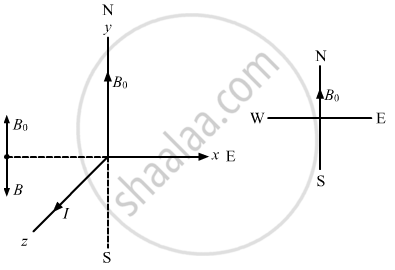Advertisement Remove all ads

# A Long, Vertical Wire Carrying a Current of 10 a in the Upward Direction is Placed in a Region Where a Horizontal Magnetic Field of Magnitude 2.0 × 10−3 T Exists from South to North. - Physics

Short Note

A long, vertical wire carrying a current of 10 A in the upward direction is placed in a region where a horizontal magnetic field of magnitude 2.0 × 10−3 T exists from south to north. Find the point where the resultant magnetic field is zero.

Advertisement Remove all ads

#### Solution

Given:
Uniform magnetic field, B0 = 2.0 × 10−3 T (From south to north)
To make the resultant magnetic field zero, the magnetic field due to the wire should be of the same magnitude as B0 and in the direction north to south.
The above condition will be satisfied when the required point will be placed in the west w.r.t. the wire.
Let the separation of the point from the wire be d.The magnetic field due to current in the wire is given by

$B = \frac{\mu_0 I}{2\pi d}$
From the question, B = B0.
$\Rightarrow 2 . 0 \times {10}^{- 3} = \frac{\mu_0 I}{2\pi d}$

$\Rightarrow 2 . 0 \times {10}^{- 3} = \frac{2 \times {10}^{- 7} \times 10}{d}$

$\Rightarrow d = {10}^{- 3}$ m = 1  mm

Is there an error in this question or solution?
Advertisement Remove all ads

#### APPEARS IN

HC Verma Class 11, Class 12 Concepts of Physics Vol. 2
Chapter 13 Magnetic Field due to a Current
Q 8 | Page 250
Advertisement Remove all ads

#### Video TutorialsVIEW ALL 

Advertisement Remove all ads
Share
Notifications

View all notifications

Forgot password?